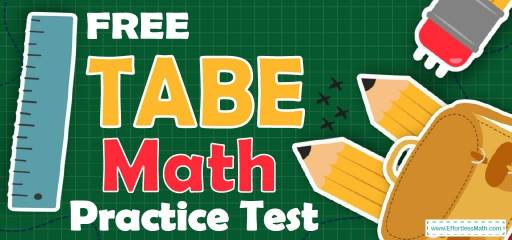# FREE TABE Math Practice TestWelcome to our FREE TABE Math practice test, with answer key and answer explanations. This practice test’s realistic format and high-quality practice questions can help you succeed on the TABE Math test. Not only does the test closely match what you will see on the real TABE, but it also comes with detailed answer explanations.

For this practice test, we’ve selected 20 real questions from past exams for your TABE Practice test. You will have the chance to try out the most common TABE Math questions. For every question, there is an in-depth explanation of how to solve the question and how to avoid mistakes next time.

Use our free TABE Math practice tests and study resources (updated for 2022) to ace the TABE Math test! Make sure to follow some of the related links at the bottom of this post to get a better idea of what kind of mathematics questions you need to practice.

## 10 Sample TABE Math Practice Questions

1- Find the average of the following numbers: $$17,13,7,21,22$$

A. 17.5

B. 17

C. 16

D. 11

2- A writer finishes 180 pages of his manuscript in 20 hours. How many pages is his average?

A. 18

B. 9

C. 6

D. 3

3- The sum of two numbers is N. if one of the numbers is 6, then three times the other number would be what?

A. $$3N$$

B. $$3(N-6)$$

C. $$3(N+6)$$

D. $$(N-2)$$

4- Which of the following fractions is the largest?

A. $$\frac{3}{4}$$

B. $$\frac{2}{5}$$

C. $$\frac{7}{9}$$

D. $$\frac{2}{3}$$

5- If $$3x-5=8.5$$, What is the value of $$5x+3$$?

A. 13

B. 15.5

C. 20.5

D. 25.5

6- What is the area of an isosceles right triangle that has one leg that measures 6 cm?

A. $$6 cm^2$$

B. $$12 cm^2$$

C. $$18 cm^2$$

D. $$36 cm^2$$

7- A shirt costing $500 is discounted $$25\%$$. After a month, the shirt is discounted another $$15\%$$. Which of the following expressions can be used to find the selling price of the shirt? A. (500)(0.60) B. (500)-500(0.40) C. (500)(0.25)-(200)(0.15) D. (500)(0.75)(0.85) 8- Which of the following points lies on the line with equation $$3x + 5y = 7$$ ? A. $$(2,1)$$ B. $$(-1,2)$$ C. $$(-2,2)$$ D. $$(2,2)$$ 9- $$8ab−5ab=$$ ? A. ab B. 3ab C. 2ab D. 5ab 10- $$8\%$$ of ___ $$= 64$$ A. 1,200 B. 1,000 C. 900 D. 800 ## Best TABE Math Prep Resource for 2022 ## Answers: 1- C $$\frac{17+ 13+7+21+22}{5}=\frac{80}{5}=16$$ 2- B $$180÷20=9$$ 3- B $$x + y = N → x = 6 → 6 + y = N → y = N – 6 → 3y = 3(N – 6)$$ 4- C $$A. \frac{3}{4}=0.75$$ $$B. \frac{2}{5}=0.4$$ $$C. \frac{7}{9}=0.77$$ $$D. \frac{2}{3}=0.66$$ 5- D $$3x-5=8.5→3x=8.5+5=13.5→x=\frac{13.5}{3}=4.5$$ Then; $$5x+3=5 (4.5)+3=22.5+3=25.5$$ 6- C First, draw an isosceles triangle. Remember that the two sides of the triangle are equal. Let put a for the legs. Then: a = 6 ⇒ area of the triangle is: $$\frac{1}{2} (6 × 6) = \frac{36}{2}= 18 \space cm^2$$ 7- D To find the discount, multiply the number by ($$100\%$$ – rate of discount). Therefore, for the first discount we get: (500)($$100\%$$ – $$25\%$$) = (500)(0.75) For the next $$15\%$$ discount: (500)(0.75)(0.85) 8- B Plug in each pair of numbers in the equation: $$3x + 5y = 7$$ $$(2,1): 3 (2) + 5 (1) = 11$$ $$(–1,2): 3 (–1) + 5 (2) = 7$$ $$(–2,2): 3 (–2) + 5 (2) = 4$$ $$(2,2): 3 (2) + 5 (2) = 16$$ Choice B is correct. 9- B $$8ab−5ab=3ab$$ 10- D 8 $$\frac{8}{100}×x=64$$ $$\frac{8x}{100}=64$$, $$x=\frac{6400}{8}=800$$ Looking for the best resource to help you succeed on the TABE Math test? ## The Best Book to Ace the TABE Test ## More from Effortless Math for TABE Test … ### Want to have a review of the top TABE math practice questions? If yes, do not miss the Top 10 TABE Math Practice Questions! ### Need a reliable math course to ensure your success in the TABE math test? We guarantee your success!: The Ultimate TABE Math Course (+ FREE Worksheets & Tests) ### Do you know what the math section of the TABE test includes? If you need more information about this, do not miss our article: What Kind of Math Is on the TABE Test? ## The Perfect Prep Booksfor the TABE Math Test ## Have any questions about the TABE Test? Write your questions about the TABE or any other topics below and we’ll reply! ## Related to This Article ### More math articles ### What people say about "FREE TABE Math Practice Test - Effortless Math: We Help Students Learn to LOVE Mathematics"? No one replied yet. X 52% OFF Limited time only! Save Over 52% SAVE$40

It was $76.99 now it is$36.99